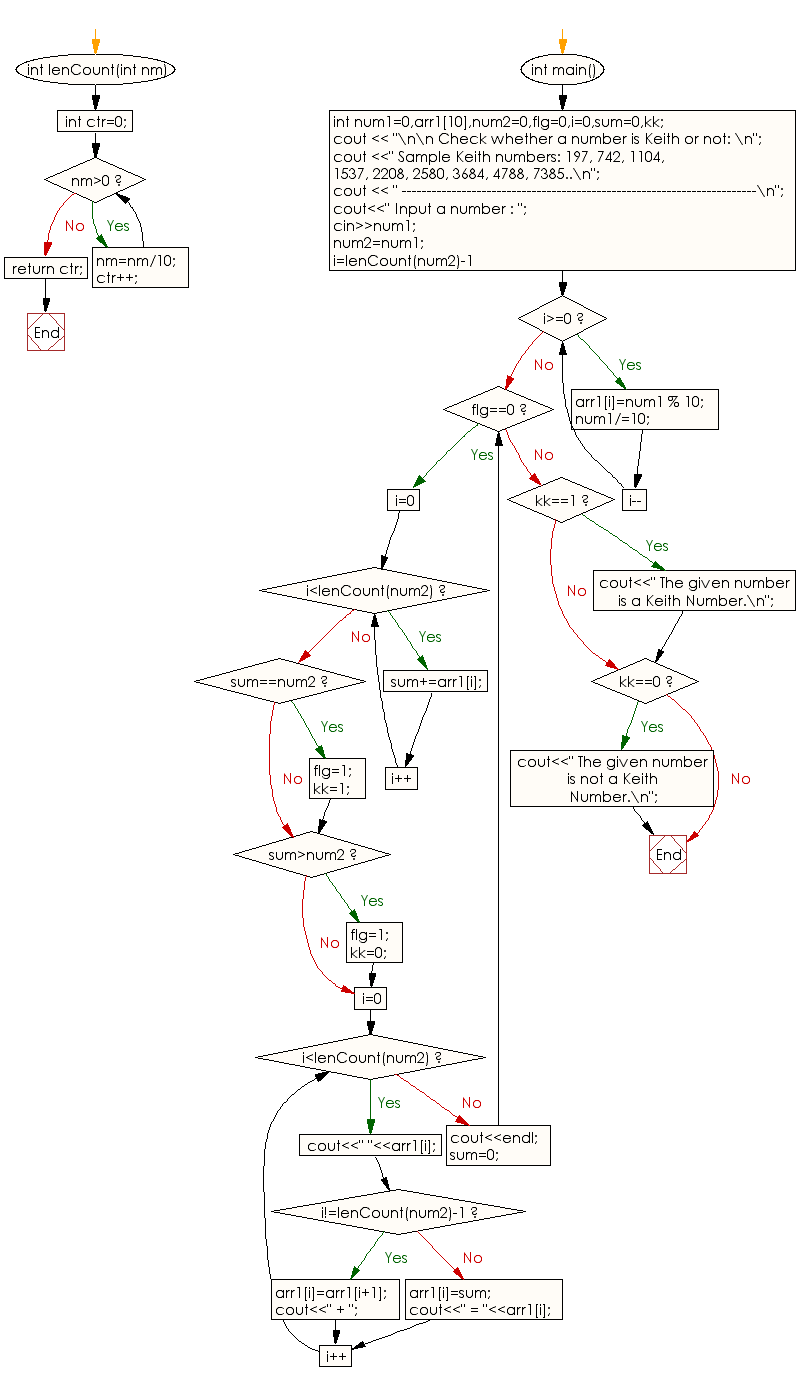﻿ C++ : Check if a number is Keith or not (with explanation)

# C++ Exercises: Check if a number is Keith or not

## C++ Numbers: Exercise-41 with Solution

Write a C++ program to check if a number is Keith or not (with explanation).

Sample Output:
Input a number : 197
1 + 9 + 7 = 17
9 + 7 + 17 = 33
7 + 17 + 33 = 57
17 + 33 + 57 = 107
33 + 57 + 107 = 197
The given number is a Keith Number.

Sample Solution:

C++ Code:

``````#include<bits/stdc++.h>
using namespace std;

int lenCount(int nm)
{  int ctr=0;
while(nm>0)
{
nm=nm/10;
ctr++;
}
return ctr;
}
int main()
{
int num1=0,arr1,num2=0,flg=0,i=0,sum=0,kk;
cout << "\n\n Check whether a number is Keith or not: \n";
cout <<" Sample Keith numbers: 197, 742, 1104, 1537, 2208, 2580, 3684, 4788, 7385..\n";
cout << " -----------------------------------------------------------------------\n";
cout<<" Input a number : ";
cin>>num1;
num2=num1;
for(i=lenCount(num2)-1;i>=0;i--)
{
arr1[i]=num1 % 10;
num1/=10;
}
while(flg==0)
{
for(i=0;i<lenCount(num2);i++)
sum+=arr1[i];
if(sum==num2)
{
flg=1;
kk=1;
}
if(sum>num2)
{
flg=1;
kk=0;
}
for(i=0;i<lenCount(num2);i++)
{
cout<<" "<<arr1[i];
if(i!=lenCount(num2)-1)
{arr1[i]=arr1[i+1];
cout<<" + ";}
else
{arr1[i]=sum;
cout<<" = "<<arr1[i];}
}
cout<<endl;
sum=0;
}
if(kk==1)
{
cout<<" The given number is a Keith Number.\n";
}
if(kk==0)
{
cout<<" The given number is not a Keith Number.\n";
}
}
``````

Sample Output:

``` Check whether a number is Keith or not:
Sample Keith numbers: 197, 742, 1104, 1537, 2208, 2580, 3684, 4788, 7385..
-----------------------------------------------------------------------
Input a number : 197
1 +  9 +  7 = 17
9 +  7 +  17 = 33
7 +  17 +  33 = 57
17 +  33 +  57 = 107
33 +  57 +  107 = 197
The given number is a Keith Number.
```

Flowchart:C++ Code Editor:

What is the difficulty level of this exercise?

﻿

## C++ Programming: Tips of the Day

Why does the C++ map type argument require an empty constructor when using []?

This issue comes with operator[]. Quote from SGI documentation:

data_type&operator[](constkey_type& k) - Returns a reference to the object that is associated with a particular key. If the map does not already contain such an object, operator[] inserts the default object data_type().

If you don't have default constructor you can use insert/find functions. Following example works fine:

```myMap.insert(std::map<int, MyClass>::value_type ( 1, MyClass(1) ) );
myMap.find( 1 )->second;
```

Ref: https://bit.ly/3PQBRJi

We are closing our Disqus commenting system for some maintenanace issues. You may write to us at reach[at]yahoo[dot]com or visit us at Facebook